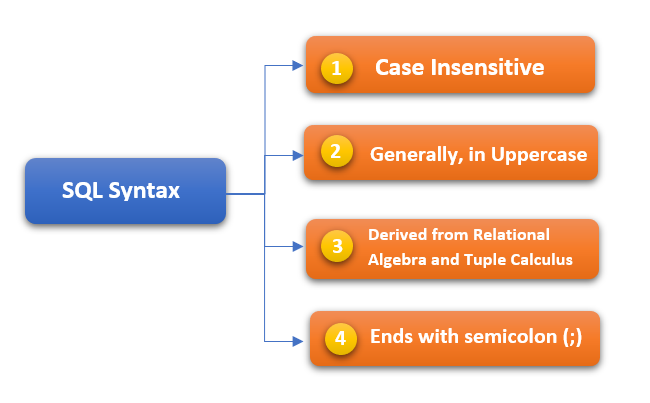# SQL Syntax

Learning Objective: In this tutorial, you will learn about SQL Syntax.

SQL language follows some set of rules and guidelines called syntax. The aim of this tutorial is to give you basic idea about the SQL syntax.

All the SQL statements start with any of the keywords like SELECT, DELETE, UPDATE, ALTER, DROP, etc. and each statement ends with a semicolon (;).### SQL Syntax Important Points

1. SQL statement are case insensitive that means in SQL, SELECT and select both are same. Whereas, MySQL makes difference in table names. So, while working in MySQL, you need to keep this in mind and have to provide exact name as they are in database.
2. Generally, SQL keywords are written in uppercase.
3. SQL syntax is derived from the relational algebra and tuple relational calculus.
4. Each SQL statement ends with semicolon (;). Semicolon are generally used to separate two SQL statement from each other where more than one statement is used.

### SQL Syntax Example

Following are the most commonly used SQL syntaxes.

All the SQL syntaxes are mentioned here are tested in MySQL database.

### SQL SELECT Statement

```SELECT column1, column2....columnN
FROM   table_name;
```

### SQL DISTINCT Clause

```SELECT DISTINCT column1, column2....columnN
FROM   table_name;
```

### SQL WHERE Clause

```SELECT column1, column2....columnN
FROM   table_name
WHERE  CONDITION;
```

### SQL AND/OR Clause

```SELECT column1, column2....columnN
FROM   table_name
WHERE  CONDITION-1 {AND|OR} CONDITION-2;
```

### SQL IN Clause

```SELECT column1, column2....columnN
FROM   table_name
WHERE  column_name IN (val-1, val-2,...val-N);
```

### SQL BETWEEN Clause

```SELECT column1, column2....columnN
FROM   table_name
WHERE  column_name BETWEEN val-1 AND val-2;
```

### SQL LIKE Clause

```SELECT column1, column2....columnN
FROM   table_name
WHERE  column_name LIKE { PATTERN };
```

### SQL ORDER BY Clause

```SELECT column1, column2....columnN
FROM   table_name
WHERE  CONDITION
ORDER BY column_name {ASC|DESC};
```

### SQL GROUP BY Clause

```SELECT SUM(column_name)
FROM   table_name
WHERE  CONDITION
GROUP BY column_name;
```

### SQL COUNT Clause

```SELECT COUNT(column_name)
FROM   table_name
WHERE  CONDITION;
```

### SQL HAVING Clause

```SELECT SUM(column_name)
FROM   table_name
WHERE  CONDITION
GROUP BY column_name
HAVING (arithmetic function condition);
```

### SQL CREATE TABLE Statement

```CREATE TABLE table_name(
column1 datatype,
column2 datatype,
column3 datatype,
.....
columnN datatype,
PRIMARY KEY( one or more columns )
);
```

### SQL DROP TABLE Statement

```DROP TABLE table_name;
```

### SQL CREATE INDEX Statement

```CREATE UNIQUE INDEX index_name
ON table_name ( column1, column2,...columnN);
```

### SQL DROP INDEX Statement

```ALTER TABLE table_name
DROP INDEX index_name;
```

### SQL DESC Statement

```DESC table_name;
```

### SQL TRUNCATE TABLE Statement

```TRUNCATE TABLE table_name;
```

### SQL ALTER TABLE Statement

```ALTER TABLE table_name {ADD|DROP|MODIFY} column_name {data_ype};
```

### SQL ALTER TABLE Statement (Rename)

```ALTER TABLE table_name RENAME TO new_table_name;
```

### SQL INSERT INTO Statement

```INSERT INTO table_name( column1, column2....columnN)
VALUES ( value1, value2....valueN);
```

### SQL UPDATE Statement

```UPDATE table_name
SET column1 = value1, column2 = value2....columnN=valueN
[ WHERE  CONDITION ];
```

### SQL DELETE Statement

```DELETE FROM table_name
WHERE  {CONDITION};
```

### SQL CREATE DATABASE Statement

```CREATE DATABASE database_name;
```

### SQL DROP DATABASE Statement

```DROP DATABASE database_name;
```

### SQL USE Statement

```USE database_name;
```

### SQL COMMIT Statement

```COMMIT;
```

### SQL ROLLBACK Statement

`ROLLBACK;`

Summary: In this tutorial, you have got some basic idea about SQL Syntax.